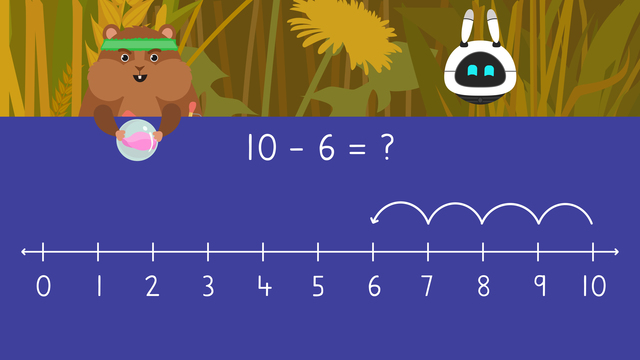# Using Number Lines to SubtractRate this video

Be the first to rate this video!

The authorTeam Digital
Using Number Lines to Subtract
CCSS.MATH.CONTENT.1.OA.A.1

## Information about the videoUsing Number Lines to Subtract

### In this video on 1st Grade Subtraction with Number Lines

Mr. Squeaks built a bowling alley so he and Imani could play a game. Mr. Squeaks starts by rolling the ball down the lane at the ten pins. He knocks down six. He wants to know how many pins are still standing, but isn’t sure how to figure it out. In this video, Mr. Squeaks and Imani learns all about using a number line to subtract.

### Subtraction with Number Lines

Closed number lines help us see the numbers as we take things away, or subtract. They already have numbers written out for us to use. We always move to the left, or backward, when we subtract on a number line. We subtract with hops on a line to show our work.Let's practice with the example eighteen minus two. Find the first number, eighteen, and mark it on the number line.Then, look at the second number, two, and count two spaces to the left, or backward. After counting, we landed on sixteen.So sixteen is our answer! We write sixteen next to the equal sign. Eighteen minus two equals sixteen!### Summary of Steps: Subtraction with Number Lines

• Start by finding the first number in the problem and marking it on the closed number line.

• Then, look at the second number in the problem and count this number of spaces to the left, or backward.

• The number we land on is our answer!

• Finally, write the answer next to the equal sign.

### Teaching Number Lines for Subtraction Strategies: Math Activities

Have you practiced subtraction with number lines worksheets yet? On this website, you can also find 1st grade subtraction worksheets with number lines and exercises.

### TranscriptUsing Number Lines to Subtract

Mr. Squeaks built a bowling alley for Imani and himself! Mr. Squeaks is up first. He rolls the ball toward the ten pins. Mr. Squeaks knocked over six pins! He wants to know how many pins are still standing. Let's help Mr. Squeaks by "Using Number Lines to Subtract". Closed number lines help us see the numbers as we take things away, or subtract. They already have numbers written out for us to use. We always move to the left, or backward, when we subtract on a number line. We draw hops, or jumps, on the line to show our work. Let's practice with the example eighteen minus two. Find the first number, eighteen, and mark it on the number line. Then, look at the second number, two, and count two spaces to the left, or backward. Let's count back together one, two! We landed on, sixteen, so sixteen is our answer! Write sixteen here next to the equal sign. Eighteen minus two equals sixteen! Now let's help Mr. Squeaks by solving ten minus six to see how many pins are left! Find the first number, ten, and mark it on the number line. Then, look at the second number, six, and count six spaces to the left. Let's count back together: one, two, three, four, five, six! We landed on, four, so four is our answer! Finally, write four here next to the equal sign. Ten minus six equals four so, Mr. Squeaks has four bowling pins left! Mr. Squeaks has one more turn to knock down the last four pins. He knocks down three of them. What is four minus three? Let's use a number line to help us subtract! What do we do first? First, we find four and mark it on the number line. What is our next step? Next, we count back three spaces since the second number is three. What number did we land on? We landed on one, so we write one next to the equal sign. Four minus three equals one, so Mr. Squeaks has one bowling pin left! Before Imani's turn, let's remember! We can use number lines to help us see the numbers we take away when we subtract. Start by finding the first number in the problem and marking it on the closed number line. Then, look at the second number in the problem and count this number of spaces to the left, or backward. The number we land on is our answer! Finally, write the answer next to the equal sign. Let's see how Imani does. Strike! “Well, I guess we don’t need a number line to know how many pins Imani has left!”